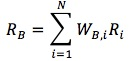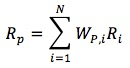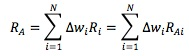### Seeing is believing!

Before you order, simply sign up for a free user account and in seconds you'll be experiencing the best in CFA exam preparation.

##### Subject 1. Active Management and Value Added
Choice of Benchmark

The benchmark:

• It should be representative of available assets.
• It can be easily replicated at low cost.
• Weights are verifiable ex ante.
• Return data are timely ex post. The return on the portfolio is based on the weights of the securities and the returns to the individual securities.
• A common example: security market index. Security market capitalization is often used to determine asset weights.

The return on the benchmark portfolio, RB:where Ri is the return on security i, and WB,i is the benchmark weight of security i.

The return on an actively managed portfolio, RP:where WP,i is the portfolio weight of security i.

The value added or active return of an actively managed portfolio:

RA = RP - RB

This is simply the difference between the return on the portfolio and the return on the benchmark portfolio.

It is ultimately driven by the differences in managed portfolio weights and benchmark weights, which are referred to as the active weights: Δwi = wP, i - wB, i

Therefore,where RAi = Ri - RB.

Positive value added is generated when positive-active-weight assets have larger returns than negative-active-weight assets. By defining individual asset active returns as the difference between the asset total return and the benchmark return, value added is shown to be positive if and only if end-of-period realized active asset returns are positively correlated with the active asset weights established at the beginning of the period.

Value added can come from a variety of active portfolio management decisions, such as security selection and asset class allocation.

Consider a portfolio of 2 asset classes, stocks and bonds:The first term is the value added from the asset allocation decision. Note that it uses the active weights and benchmark returns.

The second term is the value added from security selection. Note that it uses portfolio weights and the active returns of each asset class.

User Comment
olympria The part that is jumbled up: Positive value added is generated when positive active weight assets have larger returns than negative active weight assets. By defining individual asset active returns as the difference between the asset total return and benchmark return, value added is shown to be positive if and only if end of period realised active asset returns are positively correlated with the active asset weights established at the beginning of the period.
wuyiheng bingo